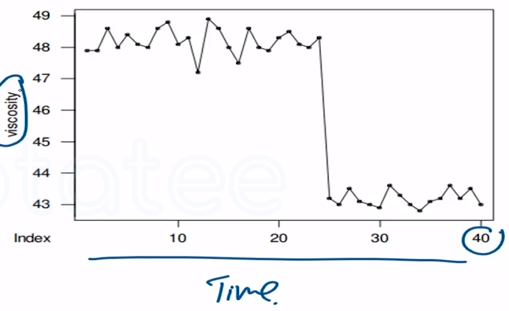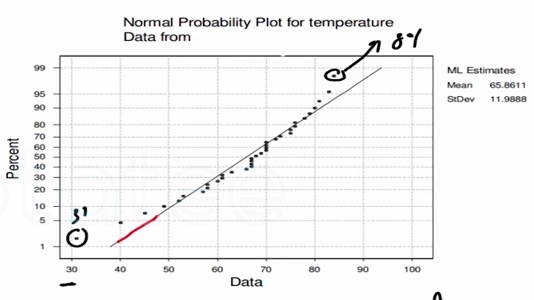Need Help?

Subscribe to Probability

###### \${selected_topic_name}
• Notes

The following data are the viscosity measurements
for a chemical product observed hourly (read down, then left
to right).

$\begin{array}{lllll}{47.9} & {48.6} & {48.0} & {48.1} & {43.0} & {43.2} \\ {47.9} & {48.8} & {47.5} & {48.0} & {42.9} & {43.6} \\ {48.6} & {48.1} & {48.6} & {48.3} & {43.6} & {43.2} \\ {48.0} & {48.3} & {48.0} & {43.2} & {43.3} & {43.5} \\ {48.4} & {47.2} & {47.9} & {43.0} & {43.0} & {43.0} \\ {48.1} & {48.9} & {48.3} & {43.5} & {42.8} \\ {48.0} & {48.6} & {48.5} & {43.1} & {43.1}\end{array}$

(a) Draw a time series plot of the dataThe following data are the joint temperatures of the
O-rings $^{\circ} \mathrm{F}$ ) for each test firing or actual launch of the space
shuttle rocket motor

$84,49,61,40,83,67,45,66,70,69,80,58,68,60,67,72,$
$73,70,57,63,70,78,52,67,53,67,75,61,70,81,76,79,$
$75,76,58,31$

Construct a normal probability plot of the O-ring joint temperature data
Does it seem reasonable to assume that O-ring joint temperature
is normally distributed?yes $\rightarrow$ normal distribution